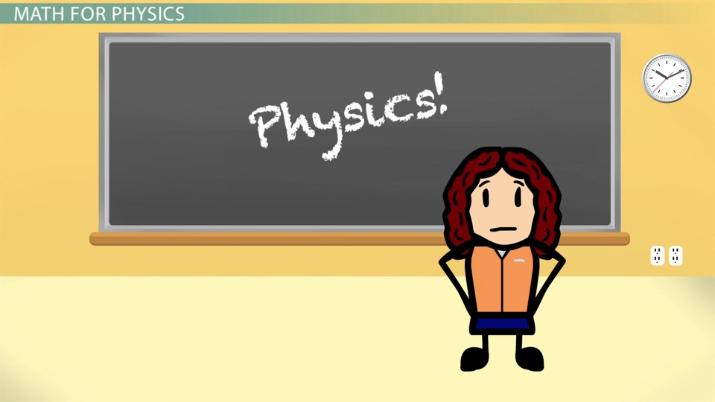# Math Review for Physics: Algebra

Lesson Transcript
Instructor: Damien Howard

Damien has a master's degree in physics and has taught physics lab to college students.

There's quite a lot of algebra you need to know to do physics. Here we'll review some of the concepts needed for rearranging equations, working with polynomials, and working with exponents.

## Math for Physics

It's no secret that many students find physics to be one of the harder classes they take. Part of the reason for this is that not only is physics very math intensive, but it also won't teach you the math you need to take it. If you're taking an algebra or calculus-based physics course, it is assumed that you already know the math necessary for it.

While we can't possibly go over every algebra concept in this lesson that might pop up in a physics course, we can review some of the algebra concepts that come up the most often in physics.An error occurred trying to load this video.

Try refreshing the page, or contact customer support.

Coming up next: Math Review for Physics: Trigonometry

### You're on a roll. Keep up the good work!

Replay
Your next lesson will play in 10 seconds
• 0:01 Math for Physics
• 0:34 Rearranging E#quations
• 2:15 Working with Polynomials
• 4:23 Exponents
• 5:45 Lesson Summary
Save Save

Want to watch this again later?

Timeline
Autoplay
Autoplay
Speed Speed

## Rearranging Equations

One of the most common things you will be doing with algebra in a physics course is rearranging physics equations. You may be doing this to simplify an equation, or you might be trying to get an unknown to one side of the equation to solve for it. Let's start by looking at the following equation.

a = b * c

Let's try to get 'b' by itself on the right side of the equal sign. So we need to get rid of that 'c.' We can do this by dividing the right side of the equation by another 'c.'

a = b * c / c

Even though we don't know what number 'c' is, we know that any number divided by itself equals one. So:

c / c = 1

Therefore, we get:

a = b * 1

Also, every number multiplied by one equals itself.

b * 1 = b

So our original equation is now the following.

a = b

However, right now this equation is incorrect. When we perform an operation to one side of the equal sign, we must also perform that same operation to the other side in order for the equation to stay true. This is necessary when performing any of the math operations. So we must divide the left side of the equal sign by 'c,' just like we did to the right. We get:

a / c = b

Now we have the correct rearranged equation with 'b' by itself on the right side of the equal sign.

## Working with Polynomials

In physics, we often work with polynomials. A polynomial is a math equation with multiple terms. For example, the polynomial a - b has the two terms of 'a' and 'b.'

Two of the most common rules for dealing with polynomials you will have to know are the distributive law and how to multiply them together.

The distributive law tells us how to multiply or divide a polynomial by a single number or variable. We do this by the following method.

a * (b + c) = ab + ac

or

(b + c) / a = b/a + c/a

The distributive law can also be done in reverse if we notice a common number or variable between terms in an equation. For instance:

ab + ac + d = a * (b + c) + d

Note that the 'd' isn't added to the parentheses because it didn't have an 'a' multiplied by it.

Now that we know how to multiply a polynomial by a single number or variable, let's look at how we multiply two polynomials together. Let's try and multiply two polynomials together in the following equation.

(a + 2)(c + 3) = ?

To multiply these two together we multiply the second polynomial by 'a' from the first plus the second polynomial again by the 2 from the first. In other words:

(a + 2)(c + 3) = a * (c + 3) + 2 * (c + 3)

We then use the distributive law to multiply the 'a' and 2 into the polynomials they are each next to. So:

(a + 2)(c + 3) = ac + 3a + 2c + 6

## Exponents

Another thing we work with a lot in physics courses are exponents. There are a multitude of useful rules that relate to exponents. Let's quickly go over them.

The zero rule states that any number raised to a power of zero is always equal to 1.

There are two one rules. The first shows that much like the zero rule, the number 1 raised to whatever power is always equal to 1.

The second states that a number raised to the first power is always equal to itself.

To unlock this lesson you must be a Study.com Member.

### Register to view this lesson

Are you a student or a teacher?